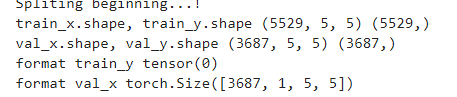# Create final image with CNN

I divided the original image (100*100) into (5.5) patches , and I took train and test percentages of the original image , but I want to find the test and train result in forma image?``````train_losses = []
# empty list to store validation losses
val_losses = []
correct = 0
total = 0
predictions =[]
actuals=[]
predictiontest=[]
# training the model
m1=0
m2=0
for epoch in range(n_epochs):
train(epoch)
plt.plot(train_losses, label='Training loss')
plt.plot(val_losses, label='Validation loss')
plt.legend()
plt.show()
output1 = model((train_x.float()),(train_x1.float()))
softmax1 = torch.exp(output1).cpu()
prob1 = list(softmax1.numpy())
predictions1 = np.argmax(prob1, axis=1)
print('Validation accuracy train: {:.4f}%'.format(float(accuracy_score(train_y, predictions1)) * 100))
output = model((val_x.float()),(val_x1.float()))
val_y = val_y.to(device)
softmax = torch.exp(output).cpu()
prob = list(softmax.numpy())
predictions = np.argmax(prob, axis=1)

total += val_y.size(0)
for i in range(predictions.size) :
if (predictions[i] == val_y[i]):
correct= correct+1

print(correct)

print('Validation accuracy test: {:.4f}%'.format(float(accuracy_score(val_y, predictions)) * 100))
j=0;
for i in range(predictions.size) :
val_y[i] = predictions[i]
plt.imshow(val_y)
conf_matrix  = confusion_matrix(val_y, predictions)
print(conf_matrix)
``````help me plz